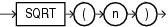# SQRT

SyntaxDescription of the illustration sqrt.gif

Purpose

`SQRT` returns the square root of `n`.

This function takes as an argument any numeric datatype or any nonnumeric datatype that can be implicitly converted to a numeric datatype. The function returns the same datatype as the numeric datatype of the argument.

Table 2-10, "Implicit Type Conversion Matrix" for more information on implicit conversion
• If `n` resolves to a `NUMBER`, then the value `n` cannot be negative. `SQRT` returns a real number.

• If `n` resolves to a binary floating-point number (`BINARY_FLOAT` or `BINARY_DOUBLE`):

• If `n` >= 0, then the result is positive.

• If `n` = -0, then the result is -0.

• If `n` < 0, then the result is `NaN`.

Examples

The following example returns the square root of 26:

```SELECT SQRT(26) "Square root" FROM DUAL;

Square root
-----------
5.09901951
```